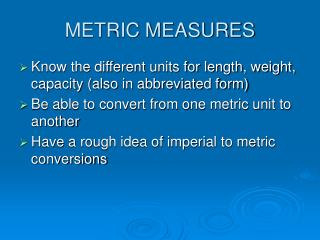# METRIC MEASURES - PowerPoint PPT PresentationDownload PresentationMETRIC MEASURES

METRIC MEASURES
Download Presentation## METRIC MEASURES

- - - - - - - - - - - - - - - - - - - - - - - - - - - E N D - - - - - - - - - - - - - - - - - - - - - - - - - - -
##### Presentation Transcript

1. METRIC MEASURES • Know the different units for length, weight, capacity (also in abbreviated form) • Be able to convert from one metric unit to another • Have a rough idea of imperial to metric conversions

2. Metric Measures mm, cm, m, km distance / length / width / height millimetres centimetres metres kilometres

3. Some rough measurements • a ruler • the height of a man • the height of a house • the distance to Marble Arch • How far can you walk in 20 minutes

4. Some common conversions to memorize • 10mm = 1cm • 100cm = 1m = 1000mm • 1000m = 1km • 1m ≈ 3feet =1 yard • 8km ≈ 5 miles • 30cm ≈ 12 inches=1foot

5. Metric Conversions  by 10  by 100  by 1000 mm cm m km x by 10 x by 100 x by 1000

6. Change these units 23cm = mm 230 96mm = cm 9.6 120cm = m 1.2 2.53m = cm 253 4500m = km 4.5 0.75km = m 750

7. Change these units using intermediate steps 1238mm = cm m 123.8 1.238 14000cm = m km 140 0.14 24500cm = m km 245 0.245

8. distance / length / width / height • I know roughly which units to use to estimate a length • I can change one unit to the next by multiplying or dividing by 10, 100 or 1000 correctly • 5 miles ≈ 8 km • The width of my little finger ≈ 1 cm • A ruler is 30cm ≈ 12 inches=1 foot

9. Metric Measures ml, cl, l, kl Capacity/volume millilitres centilitres litres kilolitres

10. Some rough measurements • a tea cup • the petrol tank of a car • a teaspoon • the bath • A fish tank

11. Some common conversions to memorize • 10ml = 1cl • 100cl = 1l = 1000ml • 1000l = 1kl • 1l = 1000cm³

12. Conversions  by 10  by 100  by 1000 ml cl l kl x by 10 x by 100 x by 1000

13. Change these units 23cl = ml 230 96ml = cl 9.6 120cl = l 1.2 2.53l = cl 253 4500l = kl 4.5 0.75kl = l 750

14. Change these units using intermediate steps 1238ml = cl l 123.8 1.238 14000cl = l kl 140 0.14 24500cl = m kl 245 0.245

15. Capacity/volume • I know roughly which units to use to estimate a volume or capacity of a container • I can change one unit to the next by multiplying or dividing by 10, 100 or 1000 correctly • 2 pints ≈ 1 litre • 1 litre = 1000cm3 • 4.5 litres ≈ 1 gallon

16. Other measures - Area Some formulas Area • Tape measure • Square units • Larger - Smaller Some units cm2, sq. cm. l x w m2, sq. m. km2, sq. km.

17. Some Areas Area of a football pitch 100m by 60m = 6000 m2 Area of a postage stamp 2cm by 3cm = 6 cm2 Area of a bedroom 3.7m by 2.5m = 9.25m2

18. Some Areas of Countries Albania:total: 28,748 sq km land: 27,398 sq km water: 1,350 sq km Angola:total: 1,246,700 sq km land: 1,246,700 sq km water: 0 sq km Afghanistan:total: 652,000 sq km land: 652,000 sq km water: 0 sq km Anguilla:total: 91 sq km land: 91 sq km water: 0 sq km

19. Other measures - speed Velocity or speed is distance per unit of time • Metres per second • Miles per hour • Kilometres per hour • Centimetres per minute Space craft Cars in Britain Cars in Europe Speed of a slow ant

20. Other measures - salaries Salaries or wages is amount of money per unit of time or even per item • £20 000 per year • £6.25 per hour • £0.10 per paper delivered • £2.60 per 100 envelopes stuffed Sometimes known as piece work

21. Other measures - Volume Volume or capacity • Amount of space in a box • Amount of air in a room • Amount of water in the pool • Amount of helium in a balloon cm3 or m3 m3 litres or m3 cm3 or m3

22. Other measures - Volume Some formulas • Tape measure • Cubic units • 1 litre is 1000 cm3 Cross section of a prism times the length of a prism l x w x h